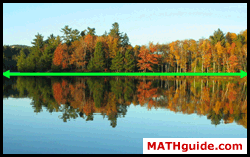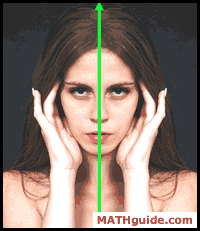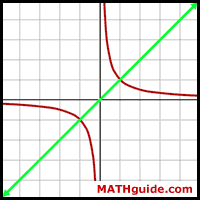Testing Relations for Symmetry
 Home > Lessons > Testing Relations for Symmetry Search | Updated June 29th, 2017
Introduction

In this section, you will learn four tests for symmetry. Here are the sections within this page:

 Symmetry Explained A description of symmetry would be this.     If a shape -- once turned, flipped, or slid -- is the same as another shape, the two shapes are said to possess symmetry. Symmetry can also be a characteristic of a single shape if it has symmetry with repsect to itself.     This picture of a scene reflecting off of a lake possesses horizontal symmetry. The top and bottom of the photo look alike.The next picture of a female model demonstrates vertical symmetry. The left and right side of the photo look like reflections off a mirror.This next picture is a graph of the equation y = 1/x. The reflection line is not horizontal or vertical. This symmetry runs along the diagonal line y = x.This next graph, which is a cube-root function, has a symmetry across the origin. The symmetry requires us to take any point on the image and reflect it over the origin. Take the point (1,1), which is a blue point above and to the right of the origin. If it is flipped directly across the origin, it lands on (-1,-1). Since (-1,-1) is a point on the curve, it demonstrates this origin (point) symmetry. All points on the cube-root curve reflect on itself.Tests for Symmetry There are four common tests for symmetry. The procedures for handling these symmetries are detailed within this table.Use the next section to see how these procedures are used. Intructional Videos Understanding symmetry and carrying out the tests for symmetry requires a bit of concentration. Watch this instructional video on symmetry to learn.ideo: Testing Relations for Symmetry Interactive Quizzes Now it is time to see if you understand the lessons above. Try this interactive quizmaster.uiz: Symmetry Check Related Lessons Try this lesson, which is closely related to the lessons above.esson: Relationsesson: Domain and Rangeesson: Inversesesson: Functionsesson: Translations / Transformations